# Types Of Matrices

In the previous article, we learned about systems of linear equations can be represented using a matrix or augmented matrix. There are many types of matrices which we are going to explore in this post.

In general matrix are referred using their order which is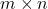where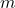is the rows and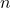is number of columns in the matrix.

If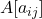is a matrix of order, then,

A = [a_{ij}]_{m \times n} = \begin{bmatrix} a_{11} & a_{12} & a_{1n}\\ a_{21} & a_{22} & a_{2n}\\ a_{m1} & a_{m2} & a_{mn}\end{bmatrix}

### Types of Matrices

There are many types of matrix in linear systems. We have listed few important ones.

• Square matrix
• Diagonal matrix
• Scalar matrix
• Unit or Identity matrix
• Null matrix
• Upper triangular
• Lower triangular matrix

There are some other types about which we shall discuss later. Let us try to know these basic matrices more.

Square Matrix

A matrix of orderwhere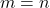is known as square matrix. For example,

A = \begin{bmatrix}1 & 4 & 9\\ 3 & 7 & 3 \\ 1 & 3 & 1\end{bmatrix}_{3 \times 3}

Note: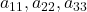are diagonal elements.

Diagonal Matrix

A square matrix with all diagonal elements as 0 is called a diagonal matrix, but the diagonal elements may or may not be zero. For example,

A = \begin{bmatrix}1 & 0 & 0\\ 0 & 7 & 0 \\ 0 & 0 & 1\end{bmatrix}_{3 \times 3}

Scalar Matrix

A diagonal matrix with equal diagonal elements are called a scalar matrix. The scalar matrix is obtained by multiplying the identity matrix with a scalar value.

\begin{aligned}
&A = \begin{bmatrix}7 & 0 & 0\\ 0 & 7 & 0 \\ 0 & 0 & 7\end{bmatrix}_{3 \times 3}\\\\
&B= \begin{bmatrix}2 & 0\\ 0 & 2\end{bmatrix}_{2 \times 2}
\end{aligned}

Unit Matrix or Identity Matrix

A square matrix with diagonal elements as 1 and all non-diagonal elements as 0 is known as a Unit or an Identity matrix. Also, note that the unit matrix is a scalar matrix in itself.

\begin{aligned}
&I_3 = \begin{bmatrix}1 & 0 & 0\\ 0 & 1 & 0 \\ 0 & 0 & 1\end{bmatrix}_{3 \times 3}\\\\
&I_2 = \begin{bmatrix}1 & 0\\ 0 & 1\end{bmatrix}_{2 \times 2}
\end{aligned}

Null Matrix

Usually no one creates null matrix, it is obtained due to some algebraic operations performed in matrices. Amatrix with all elements equal to zero is called a null matrix.

\begin{aligned}
&O_3 = \begin{bmatrix}0 & 0 & 0\\ 0 & 0 & 0 \\ 0 & 0 & 0\end{bmatrix}_{3 \times 3}\\\\
&O_2 = \begin{bmatrix}0 & 0\\ 0 & 0\end{bmatrix}_{2 \times 2}
\end{aligned}

Upper Triangular Matrix

Matrix with lower diagonals equal to zero is called an Upper triangular matrix. For example,

\begin{aligned}
&U_{3 \times 3} = \begin{bmatrix}1 & 2 & 3\\ 0 & 4 & 5 \\ 0 & 0 & 6\end{bmatrix}_{3 \times 3}
\end{aligned}

For upper triangular matrix,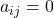, for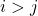and denoted by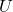.

Lower Triangular Matrix

The lower triangular matrix has its upper diagonals as zero and it is denoted by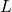.

\begin{aligned}
L_{3 \times 3} = \begin{bmatrix}1 & 0 & 0\\ 4 & 2 & 0 \\ 7 & 3 & 3\end{bmatrix}_{3 \times 3}
\end{aligned}

For lower triangular matrix,, for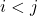and denoted by.

In the next post, we shall discuss basic row operations on matrices which are very useful in solving system of linear equations.